# Oka theorems

(diff) ← Older revision | Latest revision (diff) | Newer revision → (diff)

Theorems on the classical problems in the theory of functions of several complex variables, first proved by K. Oka between 1930 and 1950 (see ).

1) Oka's theorem on the Cousin problems: The first Cousin problem is solvable in any domain of holomorphy in; the second Cousin problem is solvable in any domain of holomorphy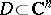that is homeomorphic to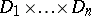, where all domains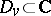, except for, possibly, one, are simply connected.

2) Oka's theorem on the Levi problem: Any pseudo-convex Riemannian domain (cf. Pseudo-convex and pseudo-concave) is a domain of holomorphy.

Originally Oka proved these theorems in dimension; in the case of arbitrary dimension, the theorems were also proved by other mathematicians.

3) The Oka–Weil theorem: Letbe a domain inand let the compact set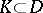coincide with its hull with respect to the algebra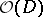of all functions holomorphic in(cf. Holomorphic envelope); then for any functionholomorphic in a neighbourhood of, and for any, a function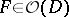can be found such that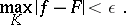This fundamental theorem in the theory of holomorphic approximation is extensively used in complex and functional analysis.

4) Oka's coherence theorem: Let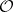be a sheaf of holomorphic functions on a complex manifold; then for any natural number, any locally finitely-generated subsheaf of the sheaf(times) is a coherent analytic sheaf (cf. Coherent sheaf).

This is one of the basic theorems of the so-called Oka–Cartan theory, which is used essentially in proving the Cartan theoremsand(cf. Cartan theorem).

How to Cite This Entry:
Oka theorems. Encyclopedia of Mathematics. URL: http://encyclopediaofmath.org/index.php?title=Oka_theorems&oldid=19092
This article was adapted from an original article by E.M. Chirka (originator), which appeared in Encyclopedia of Mathematics - ISBN 1402006098. See original article